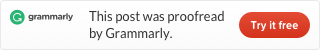Categories

# Divide and conquer algorithm (Java code and Leetcode problems)

I am trying to solve every problem of Leetcode and write blogs to record it. Now I plan to read Introduction to Algorithms and solve problems match the book contents.

## What is Divide and conquer?

Divide and conquer is a method to solve a complex problem. When we think of a problem too complex or too big, we can try to divide the problem to some easy problems or small problems. Then we try to solve these small/easy problems and combine the results to solve the complex/big problem.

Divide and conquer is easy when you can easily find out how to divide a problem. But a lot of times how to divide a problem is hard. And different problem always needs a different divide approach. So if you want to master all divide and conquer problems you need to do a lot of practices. Only this way you can build a strong understanding of the thought of divide and conquer, and get more feeling of familiarity, and master more method to divide.

Chapter 2.3.1 of Introduction to Algorithms introduces Merge sort algorithm, Chapter 4 “Divide and conquer” introduces The maximum-subarray problem and Strassen’s algorithm for matrix multiplication.

I implement these three algorithms from pseudocode from the book to Java code:

### Strassen’s algorithm for matrix multiplication Java version:

#### Simple recursive

Helper functions

Matrix split and combine functions